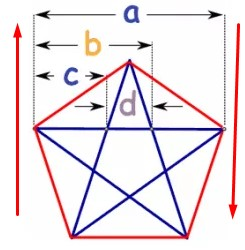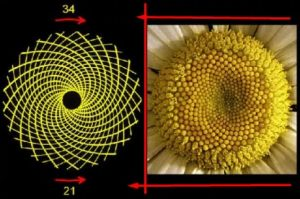# Golden Ratio: A mysterious number of the mathematicsToday we will discuss about the Golden Ratio: A mysterious number of the mathematics. First, let’s start a math with magic. Take any two numbers and sum them together. Add the second digit retained with the sum found. Now keep adding each sum to the previous number. In this way, find out till 20th number. Then, divide the 20th number by the 19th number. Then you will get the result 1.608. Regardless of starting any number, the result will be 1.608. For example, consider 5 and 2 numbers.
5+2=7; 2+7=9; 7+9=16; 9+16=25;…

Thus, the 19th and 20th numbers are available respectively – 13,153 and 21,282. This number is called the Golden Ratio. In fact, this is a witch number. Among the significant numbers of mathematical calculations, Golden Ratio is one of them. This is a proportional amount. Its value is 1.6180 and expressed by the Greek letter φ (phi). This is an irrational number. That is, it is not possible to express as a fraction of two whole numbers. A common form to publish φ is-
As it turns out, it is not possible to publish φ in a common fraction. This is an ongoing process. Another important number of mathematics is obtained (π), but it can correctly express as 22/7 fractions to several digits. For this reason, φ is called the most illogical number of mathematics.
The letter φ (Phi) derived from the initials of the Greek sculptor Phidias. Ethheni Perth was designed it based on the golden ratio in Feidius (480-430 BC). Since then the style of expressing the golden ratio to φ (phi) had been introduced. Golden Ratio also is known as Golden Section, Golden Mean, Golden Number, Devine Proportion, Devine Section, Golden Proportion, etc.

## Mathematicians and Egyptians

Many mathematicians believe that φ (phi) was familiar with the ancient Egyptians. The use of a golden Radio has observed in an Egyptian pyramid. Established in approximately 2560 BC. Euclide Elements also had a geometrical promise similar to the golden ratio. In 1509, the number became more familiar with the publication of the book “De Divina proportione” written by Luca Pacioli, father of Italian mathematician and accounting. Renaissance fame Men Leonardo DA Vinci gave geometric illustrations of the book. It is a strong proof of the fact that Vinci was very well-known with this number. Leonardo de Vinci (1452-1519) used the golden ratio in many of his works. Among them, Vitruvian Man, The Last Supper, and Mona Lisa are particularly notable.
The Golden ratio also used in the famous paintings of Michelangelo’s The Creation of Adam, Raphael’s The School of Athens, ‘Vote of Venus’, ‘The Sacred of the Last Supper’ of Battechilli, and Salvador Dalí.
Geometrical formations have a different beauty in splitting this ratio. That’s why the use of the golden ratio in different types of designs or painting in the world is noticeable. In accordance with the Golden Ratio, the geometric figure of the triangle, quadrangle, etc. can be drawn. A quadrilateral can draw in such a way that the length and breadth are in φ: 1 ratio. If the smaller quadriceps cut into φ ratio from this quadrilateral, then the smaller quadrangular arms can be found in the golden ratio with each other. In the equatorial triangle, two equal arms are in the φ: 1 ratio of land. Besides, it can divide into one φ: 1 ratio by dividing the opposite arm into one of the angled corners.
The Golden Ratio has a special geometric structure that is a ‘balanced Pentagon.’ The balanced pentagon is a bound field with five arms. Each arm is equal. It has five top points. Each top produces the same 108-degree angle and draws a five-pointed star using the angles. The star pictured in this way is called Pentagram. Notice that, a Pentagon formed between Pentagram.If the length of the Pentagon’s arm is 1 unit, the length of the stars is φ. That is, every arm of the Pentagon has a golden ear with every corner of Pentagram. There are 5 triangles in one Pentagram that have passed over each other. The figure has total triangle 35 and total quadrilateral 21. Where is the specialty of Pentagram? Apparently, the picture may look like a general geometric structure. But if you take each piece of the picture and measure it, then every Pentagram can be seen in the golden section.
The all main-golden triangle is the original triangle. Apart from each other, each line has a Golden Ratios with each other. \ Frac {a} {b} = \ frac {b} {c} = \ frac {c} {a} = φ in the image. The Pentagram is one of the world’s most recognizable symbols. Various uses of Pentagram observed in the culture of different countries. The use of Pentagram noticed in various ancient religious rituals, including magic and secret organization. The Pentagram has obtained used in more than 60 national flags.
Golden Ratio has a relationship with the Fibonacci style. This section of 0, 1, 1, 2, 3, 5, 8, 13, … is called Fibonacci Section. A number here, just like the sum of the previous two numbers. For example, 1 + 1 = 2, 2 + 1 = 3, 3 + 2 = 5, 5 + 3 = 8, 8 + 5 = 13, etc.

The Fibonacci section was discovered by Leonardo of Pissar’s Libra Abyss, written on the calculation method published in 1202. Leonardo of Pisa later became known as Fibonacci. And according to his name, the section continued named after the Fibonacci section. In the book, he used this trend to solve the problem of rabbit breeding. But Fibonacci is not considered the basic work of Fibonacci. Because of this, the mention of this section has been found in Indian math much earlier. It remains believed that the Indians discovered this section from Arabs.
At one point, there was a close relationship between the Fibonacci section and the golden ratio. The first point was noticed by German mathematician Simon Jacob, in 1564. Here one thing is noticeable that dividing the number of Fibonacci sequences by its previous number, the results found are increasingly moving towards golden ratios. For example – 8/5 = 1.6; 13/8 = 1.625; 55/34 = 1.61764; …
Fibonacci can notice in a variety of animals and plants. Because there is a close relationship between this section and the golden ratios, So it can say that nature holds Golden Ratio. There is a tendency to follow the trend in flowers, fruits, tree leaves and branches, seeds and leaves, rotating corners, birds, human bodies, music, insects, solar systems and even spiral galaxies. Nature does, however, comply with mathematical code? We do not know the answer. But this kind of nature indicates an excellent combination of math with nature. The Fibonacci Quarterly Journal has published since 1963 due to such variations of the Fibonacci series. Fibonacci and related mathematical issues are the topics of this journal’s publication.The use of φ in the design of the Parthenon has occupied a special place in the history of architecture. With the height of the width of the Parthenon, the height of the pillars from the ground has since been at the top distance with φ ratio. Moreover, the whole building can be imagined to be a golden rectangle. Greek architect Fidias designed the pattern of Parthenon in keeping with the golden ratio.Egypt’s Pyramid is another excellent example of the use of the golden ratio in architecture. The construction of the pyramid was estimated about 2570 BC. It is surprising to use the golden ratios in an ancient architecture of ancient times. However, experts are not sure whether they actually used it in an intuitive way. The Egyptians used a distance from the palm to the tip of the middle finger, which is called the cubit. This distance was equal to 52.25 centimeters. Giza’s Great Pyramid has a height of 356 cubits in height. From the center of the pyramid straight to the top point, weighs 280 cubits. One arm distance from the square floor center is 220 cubits. It has been found that the proportional figures of numbers are in different forms of golden ratios.
c/a = 356/20 = 1.618 = φ and b / a = 280/22 = 1.272 = √ φThe upper right-angled triangle founded by applying the theorem to Pythagoras, φ2 = φ + 1. Here φ, the vertical √φ and the ground 1 unit.
Golden Ratio is called the finest number of mathematics. The number is also sometimes called the heavenly number because it is more available in nature. We have seen its use in nature and art. Actually, this number does not end with greatness. That is why astronomer and mathematician Johannes Kepler said that geometry has two very valuable resources, one with Pythagorean theorem and the other is the golden ratio. We can compare the first to the gold store and the second with the precious gem.